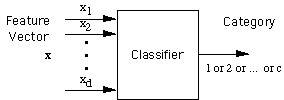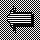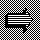# Simple ClassifiersThere are at least two ways to approach the design of a classifier:
1. Hypothesize a plausible solution and adjust it to fit the problem

2. Create a mathematical model of the problem and derive an optimal classifier
The first method is more intuitive, is frequently used in practice, and is the approach that we shall take. We start with a very simple solution, analyze its characteristics, identify its weaknesses, and complicate it only as necessary. In this section we cover the following topics:
Template matching
Minimum-distance classifiers
Metrics
Inner products
Linear discriminants
Decision boundariesBack to The ModelOn to LimitationsUp to PR for HCI